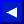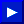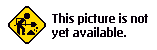The fundamental frequency defines the overall pitch of the note. Harmonics are sine waves having frequencies which are integer multiples of the fundamental frequency. A sound wave is the fundamental frequency and all of the harmonics it takes to make up that sound.In musical theory let's say that the fundamental frequency is harmonic number 1. The second harmonic has a frequency twice the fundamental frequency, the third harmonic is three times the fundamental frequency, and so on. The amounts of each harmonic present in a note give it its timbre.

An acoustic instrument, like a guitar or a violin, has a very complicated harmonic structure. In fact, the harmonic structure may vary as a single note is played. You have already played with the waveforms available in your Commodore music synthesizer. Now let's talk about how the harmonics work with the triangular, sawtooth, and rectangular waves.

A triangular wave contains only odd harmonics. The amount of each harmonic present is proportional to the reciprocal of the square of the harmonic number. In other words harmonic number 3 is 1/9 quieter than harmonic number 1, because the harmonic 3 squared is 9 (3 X 3) and the reciprocal of 9 is 1/9.

As you can see, there is a similarity in shape of a triangular wave to a sine wave oscillating at the fundamental frequency.

Sawtooth waves contain all the harmonics. The amount of each harmonic present is proportional to the reciprocal of the harmonic number. For example, harmonic number 2 is 1/2 as loud as harmonic number 1.

The square wave contains odd harmonics in proportion to the reciprocal of the harmonic number. Other rectangular waves have varying harmonic content. By changing the pulse width, the timbre of the sound of a rectangular wave can be varied tremendously.This page has been created by Sami Rautiainen. Read the small print. Last updated February 10, 2002.